# Surface Area

## Calculate the surface areas of the given basic solid shapes using standard formulae.

##### Level 1Level 2Level 3Level 4VolumeExam-StyleDescriptionHelpMore

This is level 2; Find the surface area of a variety of cuboids. The diagrams are not to scale.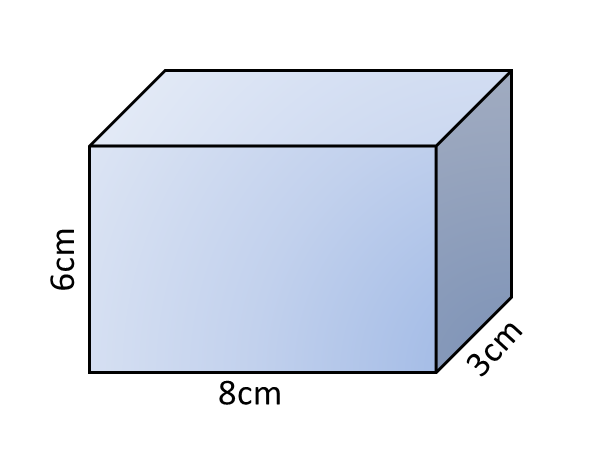1. What is the surface area of this cuboid with dimensions 6cm, 8cm and 3cm? cm22. What is the surface area of this cuboid with dimensions 14cm, 9cm and 12cm? cm2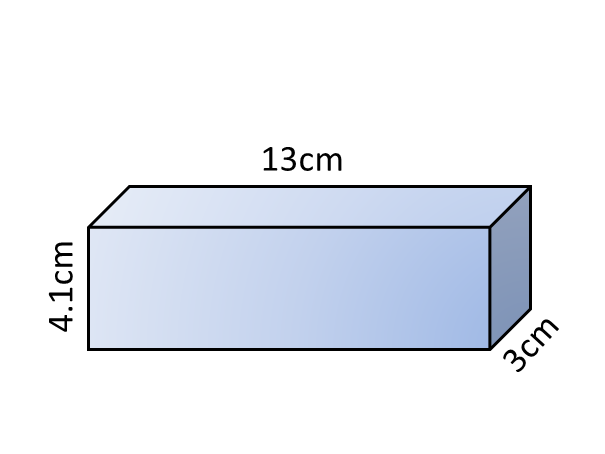3. What is the surface area of this cuboid with dimensions 4.1cm, 13cm and 3cm? cm24. What is the surface area of this cuboid with dimensions 16cm, 18cm and 9cm? cm2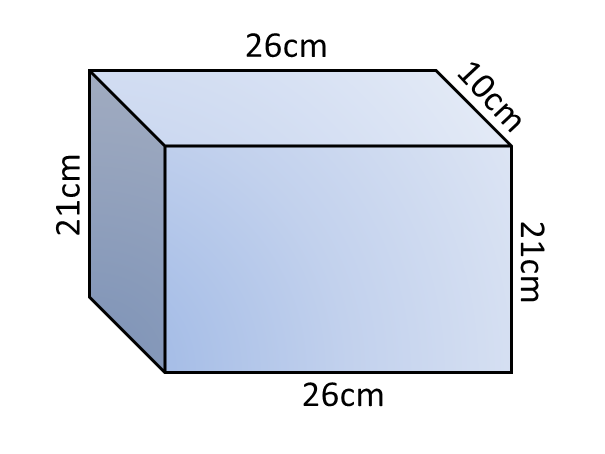5. What is the surface area of this cuboid with dimensions 21cm, 26cm and 10cm? cm2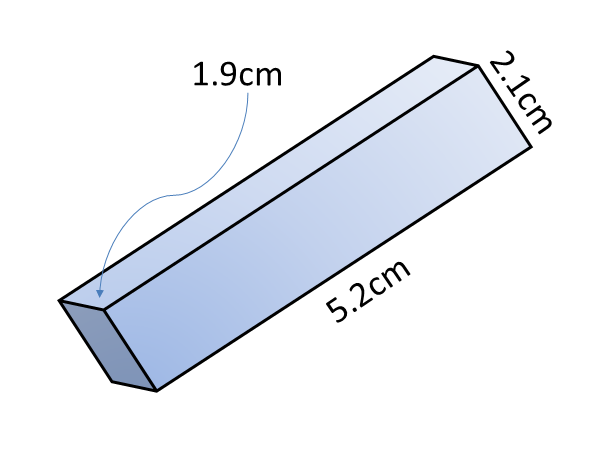6. What is the surface area of this cuboid with dimensions 1.9cm, 5.2cm and 2.1cm? cm2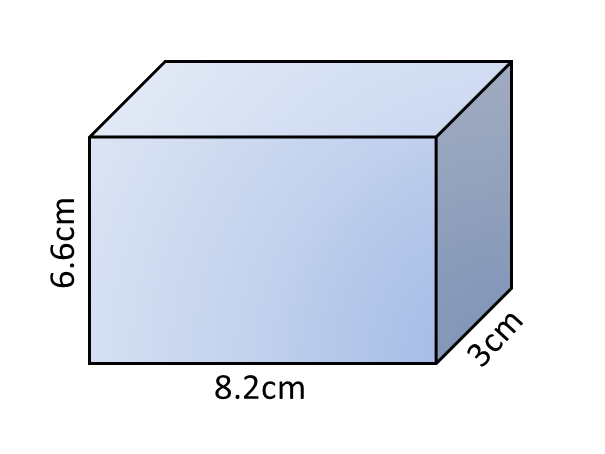7. What is the surface area of this cuboid with dimensions 6.6cm, 8.2cm and 3cm? cm28. What is the surface area of this cuboid with dimensions 88cm, 28cm and 30cm? cm2Check

## Instructions

Try your best to answer the questions above. Type your answers into the boxes provided leaving no spaces. As you work through the exercise regularly click the "check" button. If you have any wrong answers, do your best to do corrections but if there is anything you don't understand, please ask your teacher for help.

When you have got all of the questions correct you may want to print out this page and paste it into your exercise book. If you keep your work in an ePortfolio you could take a screen shot of your answers and paste that into your Maths file.

## Transum.org

This web site contains over a thousand free mathematical activities for teachers and pupils. Click here to go to the main page which links to all of the resources available.## More Activities:

Mathematicians are not the people who find Maths easy; they are the people who enjoy how mystifying, puzzling and hard it is. Are you a mathematician?

Comment recorded on the 24 May 'Starter of the Day' page by Ruth Seward, Hagley Park Sports College:

"Find the starters wonderful; students enjoy them and often want to use the idea generated by the starter in other parts of the lesson. Keep up the good work"

Comment recorded on the 16 March 'Starter of the Day' page by Mrs A Milton, Ysgol Ardudwy:

"I have used your starters for 3 years now and would not have a lesson without one! Fantastic way to engage the pupils at the start of a lesson."

#### Three Ways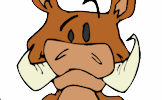Can you get to the target number by multiplying together four different one digit numbers? Can you do it in three different ways? There are nine levels to this online challenge and a virtual Transum Trophy available for each level.

There are answers to this exercise but they are available in this space to teachers, tutors and parents who have logged in to their Transum subscription on this computer.

A Transum subscription unlocks the answers to the online exercises, quizzes and puzzles. It also provides the teacher with access to quality external links on each of the Transum Topic pages and the facility to add to the collection themselves.

Subscribers can manage class lists, lesson plans and assessment data in the Class Admin application and have access to reports of the Transum Trophies earned by class members.

Subscribe

## Go Maths

Learning and understanding Mathematics, at every level, requires learner engagement. Mathematics is not a spectator sport. Sometimes traditional teaching fails to actively involve students. One way to address the problem is through the use of interactive activities and this web site provides many of those. The Go Maths page is an alphabetical list of free activities designed for students in Secondary/High school.

## Maths Map

Are you looking for something specific? An exercise to supplement the topic you are studying at school at the moment perhaps. Navigate using our Maths Map to find exercises, puzzles and Maths lesson starters grouped by topic.

## Teachers

If you found this activity useful don't forget to record it in your scheme of work or learning management system. The short URL, ready to be copied and pasted, is as follows:

Do you have any comments? It is always useful to receive feedback and helps make this free resource even more useful for those learning Mathematics anywhere in the world. Click here to enter your comments.For All:

## Description of Levels

CloseLevel 1 - Find the surface area of shapes made up of cubes.

Level 2 - Find the surface area of a variety of cuboids.

Level 3 - Find the surface area of cuboids and other composite shapes.

Level 4 - Use a formula to find the surface area of standard solid shapes.

Volume - Find the volume of basic solid shapes.

Exam Style Questions - A collection of problems in the style of GCSE or IB/A-level exam paper questions (worked solutions are available for Transum subscribers).

More on this topic including lesson Starters, visual aids, investigations and self-marking exercises.

Answers to this exercise are available lower down this page when you are logged in to your Transum account. If you don’t yet have a Transum subscription one can be very quickly set up if you are a teacher, tutor or parent.

## Surface Area Formulae

Cube: $$6s^2$$ where $$s$$ is the length of one edge.

Cuboid: $$2(lw + lh + wh)$$ where $$l$$ is the length, $$w$$ is the width and $$h$$ is the height of the cuboid.

Cylinder: $$2\pi rh + 2\pi r^2$$ where $$h$$ is the height (or length) of the cylinder and $$r$$ is the radius of the circular end.

Cone: $$\pi r(r+l)$$ where $$l$$ is the distance from the apex to the rim of the circle (sloping height) of the cone and $$r$$ is the radius of the circular base.

Cone: $$\pi r(r+\sqrt{h^2+r^2})$$ where $$h$$ is the height of the cone and $$r$$ is the radius of the circular base.

Square based pyramid: $$s^2+2s\sqrt{\frac{s^2}{4}+h^2}$$ where $$h$$ is the height of the pyramid and s is the length of a side of the square base.

Sphere: $$4\pi r^2$$ where $$r$$ is the radius of the sphere.

Prism: Double the area of the cross section added to the product of the length and the perimeter of the cross section.

###### Don't wait until you have finished the exercise before you click on the 'Check' button. Click it often as you work through the questions to see if you are answering them correctly.

Close